## General Question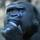# Math experts: What is the relationship between adding constant increments of pancake batter and the radius of the pancake?

Asked by gorillapaws (25747) April 20th, 2015

If you have a bunch of pancake batter and add batter to a flat surface in increments of a constant volume (say ¼ cup), it’s clear the increase in radius will become smaller with each additional ¼ cup of batter added. Is there a mathematical formula that captures this relationship? I’m using pancakes as a general example because they’re viscous, and it’s easy to visualize, but I’m mostly concerned with the abstract case.

Observing members: 0Composing members: 0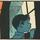The relationship is volume of pancake batter to surface area of pancake in pan. The assumption is that the batter always spreads out at a consistent rate to a set thickness.

so if a ¼ cup results in pi*r^2 size pancake, then ½ cup results in 2 (pi*r^2); ¾ cup batter equals 3(pi*r^2) size pancake.

zenvelo (35469)“Great Answer” (1) Flag as…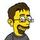A = π r^2

A / π = r^2

√ ( A / π ) = r

A is the area of the pancake, which (assuming uniform thickness) is directly proportional to the amount of batter you add,

Therefore, the radius of the pancakes will be proportional to the square root of the quantity ( Batter_used divided by Pi )

jerv (31034)“Great Answer” (4) Flag as…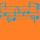In this relationship, your equation would need three dimensions if you start with an initial volume because then technically your pancake can be infinitely thin with an infinitely large radius. So, use @jerv‘s equation, but add height h:

√(A/hπ) = r

A is your independent variable, h is a parameter, and r is the dependent variable, the radius.
@jerv The relationship is not proportional; as @gorillapaws pointed out, the radius increments get smaller and smaller the bigger the pancake gets.

dxs (15101)“Great Answer” (1) Flag as…Response moderated (Off-Topic)
Response moderated (Off-Topic)
Response moderated (Off-Topic)
Response moderated (Off-Topic)
Response moderated (Off-Topic)@dxs I think there has been a misunderstanding.

1) I specifically stipulated a uniform thickness, though I did leave it unbounded with the implication that common sense would prevail and put it between zero and infinity; I have yet to even hear of any object of either infinite or zero thickness.

2) Where did I say Area was directly proportional to batter? I think you missed the part where I said, ”...square root of the quantity…”. But to rephrase;

( 1.732 – 1.414 ) < ( 1.414 – 1 ) yet ( 3 – 2 ) = ( 2 – 1 )

Watch those exponents ;)

jerv (31034)“Great Answer” (1) Flag as…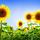Doesn’t it matter how hot the pan is?

JLeslie (59833)“Great Answer” (1) Flag as…or